Name: nishi Who is asking: Student Level: Secondary Question: how do i prove (a simply as possible) why the bond angles of a tetrahedral polygon are 109.5 degrees? *i already have two explanations that i don't understand. one is about "theory of dot products" and "vectors" and a hook-like symbol w/ a cosine, and the other has an incomprhensible diagram w/ difficult notation- PLEASE BE SIMPLE! thanks sooo much Hi Nishi, Imagine that you create the tetrahedron by joining opposite vertices on the faces of a cube as in the diagram below. To simplify the arithmetic assume that the cube has sides of length 2.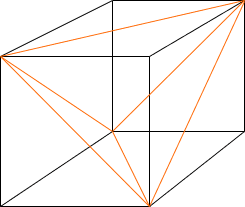Let O be the point at the center of the cube (equidistant from the eight vertices) as in the diagram below. Construct the triangle OPQ.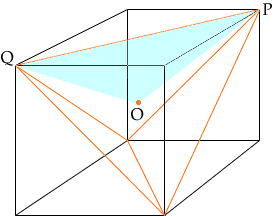Consider the triangle OPQ. Let R be the midpoint of PQ.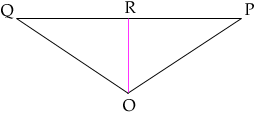Since the sided of the cube are of length 2, a use of Pythagoras' Theorem gives |PQ| =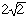. Also, since O is at the center of the cube, |OR| = 1. From the diagram the tangent of the angle ROP is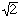. Thus angle QOP = 2 x angle ROP = 2 x arctan() = 109.5o Cheers, Harley Go to Math Central